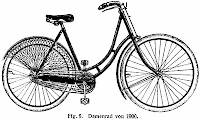# How’d They Do That Tuesday: The Physics of BicyclesI’m starting a new feature this week called “How’d they do that Tuesday.” Each week I’ll pick out some cool widget or doodad possibly even a thingamajig and take a close look at some of the physics that makes it work. So for my inaugural run, what better place to start than with a subject near and dear to my heart: Bicycles.

Bicycles are elegant machines wholly governed good old fashioned classical mechanics. No fancy quantum probability waves or relativistic space time curves here. Right now, some of the oldest and most basic laws of physics are all that we need when talking about the bicycle, starting with Newton’s second law of motion:

Mass (M) x Acceleration (A) = Force (F)

Essentially whatever energy you put into the bicycle, is what you’ll get out of it. When you push down on the pedals with whatever amount of strength, the bike will then accelerate proportionally to that, depending on the bike (and rider’s) weight. That way if I weighed 70 kg and my bike weighed 10 and I wanted to accelerate from zero to about 11 kilometers per hour (roughly 3 meters per second) all I have to do is plug in the numbers I need and I can figure out how much force I need to put into the bike.

80 kg x 3m/s^2 = 240 Newtons

Newtons are the units used to measure force, which are (kg*m)/s^2. It’s simply an algebraic way of saying 1 Newton of force would in 1 second accelerate a 1 kg object 1 meter per second faster than it was traveling before. If my bike and I combined were twice as heavy, it would take twice as much force to get the machine moving as quickly. Racing bikes are usually made of super-lightweight carbon fiber and titanium, so there’s less weight for the rider to have to push.

Gear Ratios

That’s the basic idea behind applying force to a bicycle, or any object really, but there’s a bit more to it than that. Bicycles use gear ratios to change how the force put into the bike is used to make the bike go fast. Essentially, gear ratios in a bicycle will change how far the rear wheel will turn for every turn of the pedals.

To get a good picture of how this works, imagine you’re going up a hill. No matter what gear you’re in, you’ll have to travel the same distance and ultimately use the same amount of net force, but by shifting into a lower gear, you can make it up a lot easier. When you’re in a low gear, the wheel only turns a short distance for each rotation of the pedals. This way, the force that you need to move a few centimeters forward on the road is spread over the entire turn of the pedals, making it very easy to concentrate a lot of force into a short distance.

If you tried riding up the same hill in a higher gear, when each rotation of the pedals turns the wheel two or three full rotations, you would find pedaling much more difficult. This is because while the force needed to push the bike up the same stretch of road would remain the same, it would be spread out over fewer turns of the pedals. You would need much more power (or torque) to move the pedals the same distance around.

This is in essence a simple machine in action. No matter what, a set amount of energy will be needed to climb the hill. By spreading the input of it over many turns of the pedals rather than only a few, the same amount of work can be done far more efficiently. It’s essentially the same premise that makes pulleys so efficient.

Once you’ve crested that hill, the brakes use nothing fancier than old fashioned friction to slow you down again.